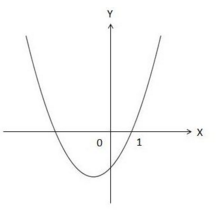Algebra Level 1The graph above is a quadratic function, $y =x^2+kx-2$ for some constant $k$. What is the solution to the quadratic inequality $x^2 + kx-2 < 0$?

Clarification: The graph intersects the $x$-axis at $x=1$.

×